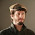### 100% Primes--While U Wait

OK, let's use the magic formula f(x) = 2x+1 to make primes:

(1) 2 is prime.
(2) 2·2+1 = 5 is prime.
(3) 2·5+1 = 11 is prime.
(4) 2·11+1 = 23 is prime--we're on a roll!
(5) 2·23+1 = 47 is prime!
(6) 2·47+1 = 95 ooops.

This gives 2 an index of 5; also, it gives 5 an index of 4, 11 an index of 3, 23 an index of 2, and 47 an index of 1.

Here's the head of the list of indices of 1, 2, ... 05204010003010001010002...

I have no idea of its properties, but they must be tricky, since their formulation must include formulating primality. Fully characterizing this sequence I should characterize as a problem of very difficult character. Have at it, my faithful legions!

Note that this protocol for framing numerical investigations can be specified for any f(x) and any propositional schema (here, "is prime").

Frame on!

1.Should it not be 3520401... ? Anyhow, I computed the first 9999 values, and 89 makes an excellent showing with a value of 6. Here are the results:
0: 8769
1: 1039
2: 149
3: 31
4: 8
5: 2
6: 1

2.Andy, we have stood each other. Your repeat is (certainly interesting enough in its own) not the same as mine.

3.My values tabulated above are frequencies. e.g. if I took your example 05204010003010001010002 I'd get
0: 14
1: 4
2: 2
3: 1
4: 1
5: 1

I just ran 9999 values instead of 23. I still think, if I 'stand it right,
1: 1, 3, 5, 15... index = 3

4.Make that 1: 1, 3, 7, 15, still index=3

1.Andy, my mistake! As to the first digit of the index sequence, I waffled, but went with the doctrinaire version where 1 is not a prime.

5.Ah, got it.